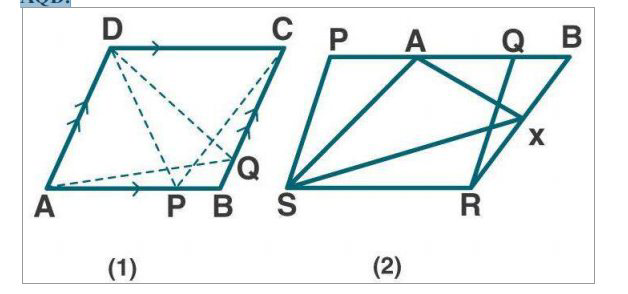# ML Aggarwal Solutions Class 9 Mathematics Solutions for Theorems on Area Exercise 14 in Chapter 14 - Theorems on Area

(a) In the figure (1) given below, ABCD is a parallelogram. P, Q are any two

points on the sides AB and BC respectively. Prove that, area of ∆ CPD = area of ∆

AQD.

(b) In the figure (2) given below, PQRS and ABRS are parallelograms and X is any

the point on the side BR. Show that area of ∆ AXS = ½ area of ||gm PQRS.(a) Given:

From fig (1)

||gm ABCD in which P is a point on AB and Q is a point on BC.

To prove:

area of ∆ CPD = area of ∆ AQD.

Proof:

∆ CPD and ||gm ABCD are on the same base CD and between the same parallels AB and

CD.

ar (∆ CPD) = ½ ar (||gm ABCD) …. (1)

∆ AQD and ||gm ABCD are on the same base AD and between the same parallels AD and

BC.

ar (∆AQD) = ½ ar (||gm ABCD) …. (2)

from (1) and (2)

ar (∆ CPD) = ar (∆AQD)

Hence proved.

(b) From fig (2)

Given:

PQRS and ABRS are parallelograms on the same base SR. X is any point on the side BR.

Join AX and SX.

To prove:

area of ∆ AXS = ½ area of ||gm PQRS

we know that, || gm PQRS and ABRS are on the same base SR and between the same

parallels.

So, ar ||gm PQRS = ar ||gm ABRS …. (1)

we know that ∆ AXS and || gm ABRS are on the same base AS and between the same

parallels.

So, ar ∆ AXS = ½ ar ||gm ABRS

= ½ ar ||gm PQRS [From (1)]

Hence proved.

Related Questions
Exercises

Lido

Courses

Teachers

Book a Demo with us

Syllabus

Maths
CBSE
Maths
ICSE
Science
CBSE

Science
ICSE
English
CBSE
English
ICSE
Coding

Terms & Policies

Selina Question Bank

Maths
Physics
Biology

Allied Question Bank

Chemistry
Connect with us on social media!